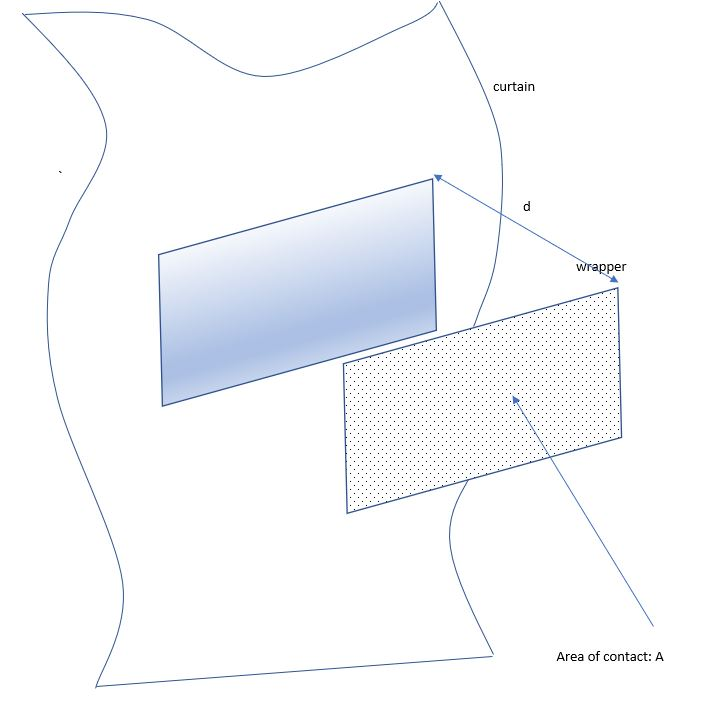# Why do chocolate wrappers stick to things

Here’s something I saw while lazily surfing the net this morning. Someone throws a candy wrapper towards the floor and it sticks to the curtain or a book cover. How long will it stick?

First, the reason this happens is because of static electricity – and this is why this rarely happens in humid climates (except inside the confines of an air-conditioned room, which is usually much drier). Why static electricity – when you eagerly unwrap a piece of chocolate, some charges jump in or out. Usually slow moving positive ions stay where they are – the electrons jump and which direction they jump in is a simple question with a complicated answer.

Clearly the answer to how long it will stick depends on how dry the air is – the mechanism for leaking the charge back between the wrapper to the curtain to the ground depends on the resistance to current flow offered by the air between the wrapper and the curtain and the capacitance of the wrapper-curtain capacitor.

Since I finished teaching “RC” circuits to a whole lot of undergraduates last month, I thought it would be fun to carry out a small calculation.

What does the system look like – let’s idealize the wrapper/curtain capacitor to the below picture.The effective area of contact is of area$A$ and separated by a distance$d$ from the curtain. This is a parallel plate capacitor, whose capacitance is (from your dim memory of high school physics)$= \frac{ \epsilon_0 A}{d}$ where$\epsilon_0$ is the permittivity of the vacuum and I have used a dielctric constant of 1 for air. Using Meter-Kilogram-Seconds-SI units, if you make the entirely reasonable assumption that$d = 1 \: mm$ and$A = 1 \; mm^2$, this translates to a capacitance of$\approx 10^{-14}$ farads. The resistivity of air (between the plates of this parallel plate capacitor) is$\rho \approx 10^{16} \Omega -m$ and again the resistance is$R = \rho \frac{d}{A}$, which approximates to$10^{19} \Omega$. The value of$R \times C \approx 10^5 secs \approx 1 \: \: day$ which is an enormously long time constant – post one day, chances are the force keeping it up (the electrical attraction) is not big enough to withstand gravity and it is likely to fall. So a candy wrapper could remain stuck for a day at least – I would bet on more since the contact area might actually be much more than$1 mm^2$ given lots of folds in the wrapper and the curtain, as well as all the sticky saliva on the wrapper from licking the candy off….reminds me I need to get back to finishing the candy!